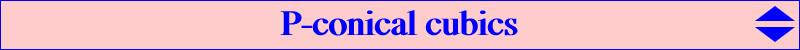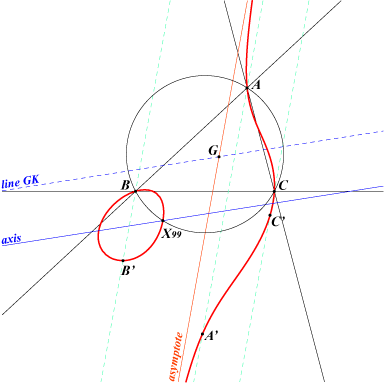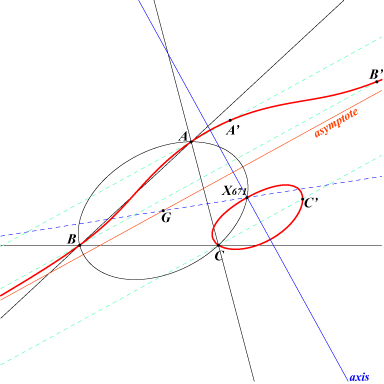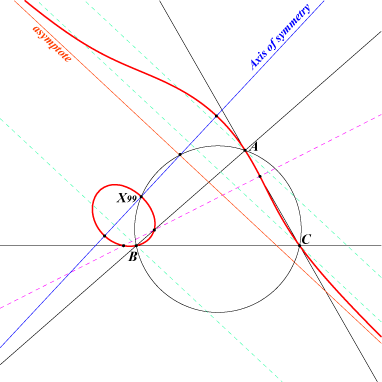This page written from an idea by Wilson Stothers and with his active cooperation   P-conical cubics generalize the class of isogonal circular non-pivotal cubics. They include the class of central non-pivotal cubics and several other classes. They arise in two apparently unconnected ways. Notation aP denotes the anticomplement of P. cP denotes the complement of P. tP denotes the isotomic conjugate of P. For fixed U, P* denotes the U-isoconjugate of P. T(P) denotes the tripolar of P. N(P) denotes the Newton line of the quadrangle formed by AB, BC, CA, T(P). nK(U,R,Q) denotes the cubic with pole U, root R passing through Q. C(P) denotes the circumconic with perspector P. Definition nK(U,R,Q) is P-conical if it includes the infinite points of C(P). Thus, a K-conical cubic is a circular cubic. Lemma 1 (1) For any R, N(R) = T(taR). (2) For any P, T(P) = N(ctP). (3) Q lies on N(P) if and only if P lies on N(Q). Of course, (2), (3) follow instantly from (1). Note that N(P) is the polar of P in the Steiner inellipse. Thus N(P) /\ N(Q) is the pole of PQ in this inellipse. U-conical nK isocubics We shall concentrate on nK(U,R,Q) which are U-conical. We usually take Q as the remaining infinite point so that Q* is the third intersection with C(U). Then the asymptotes at the infinite points of C(U) meet at this point Q* (this follows easily from Special Isocubics §1.5.1). Theorem 1 Suppose that nK(U,R,Q) is U-conical and that R is not G. Then the third infinite point on the cubic is Q the infinite point of N(R). Conversely, if Q is the infinite point of N(R), then nK(U,R,Q) is U-conical. If R = G, then nK(U,R,Q) degenerates as C(U) and the line at infinity. If C(U) is a parabola with infinite point N, then nK(U,R,Q) = cK(#N,R).   We now look at a locus which turns out to be a U-conical cubic with pole U. Theorem 2 For any line L = T(P), the locus K(U,L) = { M : the mid-point of MM* lies on L } is the U-conical cubic nK(U,R,Q), where R is at(P), so L = N(R), and Q is the infinite point of N(R). We thus have two definitions of a class of cubics, one as the class of U-conical cubics with pole U, the other as the class of loci K(U,L). The Theorems allow us to identify the points R and Q for K(U,L), and L for a U-conical nK(U,R,Q). We have a net of cubics, each member is identified uniquely by the point R. The U-conical cubic of type nK with pole U, root R Given R, let Q be the infinite point on N(R). Then K(U,N(R)) = nK(U,R,Q). If U = u:v:w and R = r:s:t, then the equation is rx(wy^2+vz^2) + sy(uz^2+wx^2) + tz(vx^2+uy^2) + K xyz = 0, where K = r(-u+v+w)+s(u-v+w)+t(u+v-w). Remarks The cubic is of type nK0 if and only if U is on N(R), i.e. R is on N(U). The cubic degenerates if R = G or U is on T(R'), R' the infinite point on T(R). The asymptote at Q is the homothetic of N(R) under h(Q*,2). Examples If U = G, we get CL031, the G-conical nK0(G,R) have R at infinity. If U = K, we get CL025, the K-conical nK0(K,R) have R on the orthic axis. If we choose Q at infinity, nK(U,R,Q) is U-conical if and only if Q is the infinite point on N(R). This is equivalent to the condition that R is on N(Q). As Q is at infinity, N(Q) = T(tQ), but this tends to obscure the general pattern that it is the N(R) which matter. *** Before looking at the general case, we take a look at cases where Q is on C(U). Let the infinite points on C(U) be Q1 and Q2. These are equal if and only if C(U) is a parabola. Then Q1=Q2 and U is the barycentric square of their common value. We are interested in real Q, so C(U) must be a hyperbola or parabola if Q = Q1. Q = Q1 distinct of Q2 By looking at the equations in Theorem 1, we see that the tangent at Q1 is the line at infinity. The tangential must then be Q2, which is then a flex. The tangent at Q2 is also the tangent to C(U) there. We then have a pencil of axial nK, each with centre Q2, and common asymptote, the above tangent. The axis is N(R), where R is the root. The root lies on N(Q1). Note that the axis is the line of mid-points of MM*. The degenerate cases arise from R = G or the barycentric quotient Q2 over Q1. Q = Q1 = Q2 We now have Q2 = Q1*, and U the barycentric square of Q1. The centre of C(U) is Q1. We know from Theorem 1 that, for any Q nK(U,R,Q) = cK(#Q1,R), and R on N(Q). The equations show that the tangent at Q1 is the line at infinity, so Q will be its tangential. When we choose Q = Q1, we get a flex at Q. We have a pencil of U-conical cubics of the form cK(#Q,R) with R on N(Q). These have centre Q. The pencil has one cubic of type cK0. This has R = T(tQ) ) /\ T(Q) - note that N(U) = T(Q). This root R is actually the tripolar centroid of Q. The class of these cK0(#Q,T(tQ) ) /\ T(Q)) with varying Q is CL029. Central non-pivotal isocubics Now suppose that we have a central non-pivotal isocubic with pole U, centre N. From Special Isocubics §3.3.1, we have the following information. (1) N is on C(U), so N* is the infinite point on NN*, (2) the root R is the complement of the isotomic conjugate of the tripole of NN*. (3) the cubic meets C(U) at its infinite points. In our notation, (3) says that the cubic is U-conical. If we compare (1) and (2) with Theorem 2, we see that our central cubic is { M : NN* bisects MM*}, and we can deduce (3). Theorem 3 If nK(U,R,Q) is central, then it is U-conical, so R is on N(Q). nK(U,R,Q) is central if and only if R = N(Q) /\ N(Q*) (unless Q = Q* or Q is on C(U)). If we fix U, we get central isocubics for R on the homothetic of cK(#G,aU), under h(G,1/4). If we fix the centre M, then U lies on T(M) and R on N(M).   Note that our class includes all central cubics. What appears to be new in general is that the cubics are loci of the form K(U,N(R)). Examples If U = K, we get CL001, cK(#G,aU) is the Simson Cubic K010. If U = G, we get CL002, cK(#G,aU) is the Tucker Cubic K015. If M = G, we get CL012. If M = O, we get CL013.   Non-pivotal isocubics with oblique symmetry We may also look for cubics with an oblique symmetry. Each is defined by a centre (at infinity) and an axis. In our calculations, we meet the line L(U,Q). If U = u:v:w, Q = l:m:n, the line has equation fx+gy+hz = 0, where f = u l (w m^2-v n^2)^2, and g, h are defined similarly. Theorem 4 If the U-conical cubic nK(U,R,Q) has an oblique symmetry, then - the centre of the symmetry is Q, - the axis of the symmetry is the parallel at Q* to the polar of Q in C(U), - the root of the cubic is R = N(Q) ) /\ L(U,Q). Note that, when Q is the infinite point of an axis of C(U) or the infinite point of an asymptote of nK0(U,U), the cubic is an axial cubic. In particular if U = K, we get CL027, the class of isogonal axial focal cubics. Our approach gives a different description of the axis of symmetry, and identifies the root algebraically. The general equation of nK(U,R,Q) with an oblique symmetry is complicated. You might want to download a text file. Cubics with fixed U and Q If we fix U, then there are four points with barycentric square equal to U. We refer to these as U1, U2, U3, U4. For U = K, we take K1 = I, and for U = G we take G1 = G. The U-conical nK with pole U passing through a fixed Q at infinity form a pencil of cubics. nK(U,R,Q) belongs to the pencil if and only if R is on N(Q). The pencil contains three degenerate cubics, with R = G or R on N(Q) and C(Q*) (see Note 1). one cubic of type nK0, with R = N(Q) ) /\ N(U) (unless U = Q). four cubics of type cK, that with node Ui has R = N(Q) ) /\ N(Ui) (unless Q = Ui). one central cubic, with R = N(Q) ) /\ N(Q*) (unless Q = Q*). one cubic with an oblique symmetry centre Q, with R = N(Q) ) /\ L(U,Q). Note 1 These give the R for which the cubic degenerates as a line and circumconic. The line being the tripolar of R and the conic the isoconjugate of this tripolar. There may be a further degenerate member. This occurs if Q is the infinite point of a cevian of one of the points Ui. Then R is the mid-point of this cevian. If C(U) is a parabola, then we may take U1 at infinity. When Q is the infinite point of any sideline of the anticevian triangle of U1, all members of the pencil are degenerate. Note 2 It would be natural to ask about cubics of type nK+. As two asymptotes meet at Q*, such a cubic would be an nK++. The algebra shows that these are precisely the central cubics. Example Here, we characterize the isogonal circular cK with node I. If U = K and U1 = I, we get CL003, with root R on N(I), Q the infinite point on N(R). Of course, some of these may coincide. For example, we have Theorem 5 The U-conical nK(U,R,Q) is a central nK0 if and only if Q is on pK(U,U).   Isotomic cubics with oblique symmetry When we choose U = G, the root of the cubic turns out to be the tripolar centroid of Q*. Suppose that Q = f:g:h. Then the axis L has coefficients the coordinates of the tripolar centroid of tQ', where Q' has barycentrics g-h:h-f:f-g. The real asymptote of the cubic always contains G. If we vary Q, then R lies on a sextic. This decomposes into the union of the medians and a cubic. This cubic is the image of the Tucker Cubic K015 under the homothety h(G,1/2).Examples of isotomic cubics with oblique symmetry nK(G, X1641, X523) passing through X(99), X(523) (figure) nK(G, X1644, X514) passing through X(190), X(514) nK(G, X1651, X525) passing through X(525), X(648)Isotomic axial cubics the cubic has an axis of symmetry if and only if Q is the infinite point of one of the axes of the Steiner ellipse. In such case, the axis of symmetry is the parallel at X(671) to the other axis of the Steiner ellipse.Isogonal axial cubics The formulae for R can be used for any pole U. For U = K we get members of CL027. You get an axial isogonal circular cubic for each infinite Q. Such cubic is always a focal cubic. For example, with Q = X(512), we get the Steiner axial cubic nK(K, R, X512) with R on the line GX(39) having first barycentric coordinate : a^4[b^4+c^4-a^2(b^2+c^2)]^2-b^2c^2(a^4-b^2c^2)^2. The real asymptote is X(39)X(512), the axis is X(99)X(511).